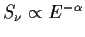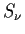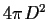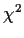Next: Structure of the Spectral Up: Spectral Analysis Previous: Notes on IPC and

## Model Spectra for Spectral-Fitting Program

For on-line information type: cl> help using_spectral

The models provided are:

• abs - absorption of x-rays by neutral gas with solar abundances.

 parameter 1 = logarithmic value of Galactic NH in units of cm-2 parameter 2 = logarithmic value of Intrinsic NH in units of cm-2 parameter 3 = redshift of source

If only one parameter is given, it is assumed to be the Galactic NH.

If only two parameters are given, they are assumed to be the Intrinsic NH and the redshift.

• pow - power law

 parameter 1 = normalization - logarithmic value of the flux density at 1 keV in units of keV cm-2 s-1 keV-1 parameter 2 = energy index defined as, whereis the flux density

• bre - thermal bremsstrahlung (exponential plus gaunt factor)

 parameter 1 = normalization - logarithmic value of the flux density at 1 keV in units of keV cm-2 s-1 keV-1 parameter 2 = temperature in the source frame in KeV

• exp - pure exponential

 parameter 1 = normalization - logarithmic value of the flux density at 1 keV in units of keV cm-2 s-1 keV-1 parameter 2 = temperature in the source frame in KeV

• bla - black body

 parameter 1 = normalization - logarithmic value of the flux density at 1 keV in units of keV cm-2 s-1 keV-1 parameter 2 = temperature in the source frame in keV

• ray - optically thin thermal spectrum with metallic lines (Raymond code)

 parameter 1 = normalization - logarithmic value of the ratio of the volume emission measure to, where D is the distance to the target. Units of the normalization are cm-5. parameter 2 = temperature in the source frame in keV parameter 3 = abundance table c - cosmic m - Meyer parameter 4 = percentage of heavy elements (100 is the solar value)

• line - single gaussian line model

 parameter 1 = normalization - logarithmic value of the normalization of the gaussian in units of keV cm-2 s-1. parameter 2 = line centroid in keV parameter 3 = FWHM of the line in keV

For detailed information on model parameters, please refer to the science specifications in xspectraldoc\$spectral.(tex,dvi).

The spectral model and its parameters are passed to the fitting tasks as:

absorption(params)*model(params)

More than one absorption and more than one spectral model can be combined in the following ways:

absorption1(params)*model1(params)+absorption2(params)*model2(params)...

or

absorption(params)*(model1(params)+model2(params)...+modelN(params))

Some basic rules for writing a model description:

• Commas or spaces separate parameters
• Lo:hi should be given when a parameter should vary within these boundaries. A single number indicates a fixed parameter value.
• There are no implicit parameters (i.e., all parameters must be specified). The exception is the normalization. For a single model the normalization does not need to be specified and will be calculated. For a combination of models, however, either a range or a specific value for the normalization must be given for all but the first model. The normalization in the first model will be calculated as before. A single value for the normalization (i.e., fixed normalization) will force the program to use that value.
• Normalization for models 2 ... N are relative to that of model 1. To specify a fixed normalization for model 2, 50% that of model 1, the syntax is:

abs(18:20) * ( power(0:2) + brems ( log(0.5), 0:20) )

NOTE: When different spectral models with different units for the normalization are combined, the relative normalization of the second model can be quite large.

For example: ray(1:2,c,100)+pow(11:13,1:2)

specifies a power-law model with a flux of approximately 1% of the flux in the Raymond model.

Note: The program fit starts at the midpoint of the parameter range specified, then changes the parameters to determine the direction in whichis decreasing and heads in that direction to find the parameter values that give the minimum. If the initial parameter range is large, only part of the parameter space may be tested, and the program can settle into a secondary minimum. The real minimum can be missed. Choose parameter limits carefully.

Examples:

1.
abs(20:21)*pow(1:2)

to describe a power law model spectrum, with energy index varying between 1 and 2, and a galactic absorption varying between 1020 and 1021 cm-2.

2.
abs(20:21,23,1.0)*(pow(1:2)+pow(log(0.5):log(0.6),1.5)

for two power law models, and one absorption applied to both of them. The galactic absorption can vary between 1020 and 1021 cm-2, the intrinsic absorption is fixed at 1023, for an object at z=1. The energy index of the first power law model can vary between 1 and 2, with the best fit normalization calculated by the program. The second power law has a fixed index of 1.5, and its normalization is allowed to vary between 50 and 60 % of the first one.

3.
abs(20:21)*pow(1:2)+abs(21:22)*pow(0.1,2:3)

to describe two power law models, each with galactic absorption in two different ranges.Next: Structure of the Spectral Up: Spectral Analysis Previous: Notes on IPC and
rsdc@cfa.harvard.edu
1998-06-10